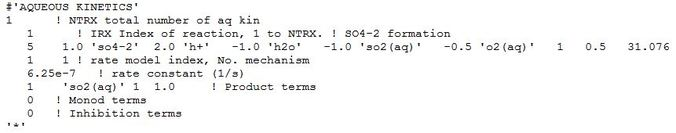0# Questions on aqueous kinetics, Sample Problem 10.

Hello everybody,

I have some questions concerning the representation of aqueous kinetics reactions and especially the sample problem 10 from the Toughreact V3-OMP description.

1) In table 1.10-2 (p. 75 in the pdf) the value for V_m^Fe is given as 15.0e-12, while in the example of the chemical.inp file (p. 80) it is written as 3.0e-12. Does anybody know which one is correct?

2) The units for the Vm rate constant values in that particular table are specified as s^-1, while in the input file they are denoted as mol/kg*s, which should make more sense from my point of view. Which one is correct?

3) At a different example (no. 9, p. 67), the unit for the rate constant is given as mass/L*s, but how is (or can be) this related to reactions where different chemical species are produced?

4) I tried to use aqueous kinetics for the oxidation of SO2(aq) to SO4-2 and added the following input:I tried to include the affinity term (fG) as shown, but the simulation does not work. If I exclude the specific part in record Akin-4, the simulation runs. Has anybody had similar problems?

5) The value 31.076 in record Akin-4 is the log of the equilibrium constant for that reaction calculated from the regression coefficients from the data base at the prevailing temperature of 66°C. Although my actual simulation is isothermally treated, how could the K value be included for varying temperatures? Or is this value limited to a temperature-independent treatment?